5G时代下一个聚合的编程学习网

# 1、隐马尔可夫简介

​ 在很多人看来，HMM、CRF这种传统算法很low！似乎在深度学习大行其道的年代，经典算法已经成为很多人眼中的鸡肋，只有AI才是牛掰。事实真的是这样吗？不可否认，深度学习的发展让人欣喜，其未来前景也让人期待。但是深度学习之所以火热，很大程度上是因为大数据与算力。那么在我们要解决的问题中，都有大数据支持吗？很遗憾，实际生活中并不都是这样的。

​ 如果你深入分析近年来在深度学习上的发展，在各种trick层出不穷的同时，我们也不难看到传统经典算法的影子。马尔可夫链在统计学中是非常著名的模型，隐马尔可夫在工业应用中非常广泛。实际上这种强假设模型仍然有非常大的市场。

## 1.1 隐马尔可夫定义

• 隐藏的马尔可夫链随机生成的状态的序列，称为状态序列
• 每个状态生成一个观测，而由此产生的观测的随机序列，称为观测序列

## 1.2 隐马和马的区别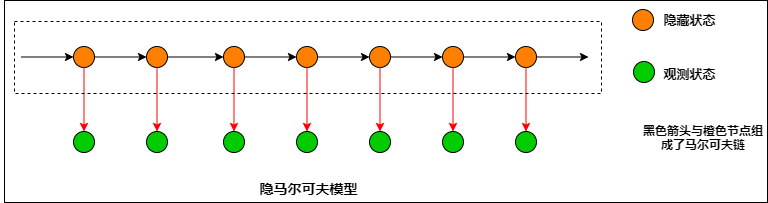## 1.3 隐马尔科夫模型的基本假设

[P left( i_{t}|i_{t-1},o_{t-1},cdots,i_{1},o_{1} ight)=P left( i_{t}|i_{t-1} ight), quad t=1,2, cdots,T
]

[P left( o_{t} | i_{T},o_{T}, i_{T-1},o_{T-1},cdots,i_{t+1},o_{t+1},cdots,i_{t},o_{t},i_{t-1},o_{t-1},cdots,i_{1},o_{1} ight)=P left( o_{t} | i_{t} ight)
]

## 1.4 符号说明

• (Q= left{ q_{1},q_{2},cdots,q_{N} ight}) 所有状态的集合；

• (V= left{ v_{1},v_{2},cdots,v_{M} ight}) 所有观测的集合；

• (I = left{ i_{1},i_{2},cdots,i_{T} ight}) 长度为(T)的隐状态序列；

• (O = left{ o_{1},o_{2},cdots,o_{T} ight}) 对应的观测序列；

• (A = left [ a_{ij} ight ]_{N imes N}) 状态转移概率矩阵；

• (a_{ij}=Pleft ( i_{t+1}=q_{j} | i_{t}=q_{i} ight ),quad i=1,2,cdots ,N;quad j=1,2,cdots ,N)

(t)时刻处于状态(q_{i})，下一时刻处于(q_{j})的概率。

• (B=left [ b_{j}left ( k ight ) ight ]_{N imes M}) 观测的概率矩阵；

• (b_{j}left ( k ight ) = Pleft ( o_{t}=v_{k} | i_{t}=q_{j} ight ),quad k=1,2,cdots ,M; quad j=1,2,cdots ,N)

​ 在(t)时刻处于状态(q_{j})下生成观测(v_{k})的概率；

• (Pi=(pi_{1},pi_{2},cdots,pi_{N})) 初始状态概率向量。

[lambda = left( A,B,Pi ight)
]

# 2、隐马尔可夫案例

## 2.1 案例描述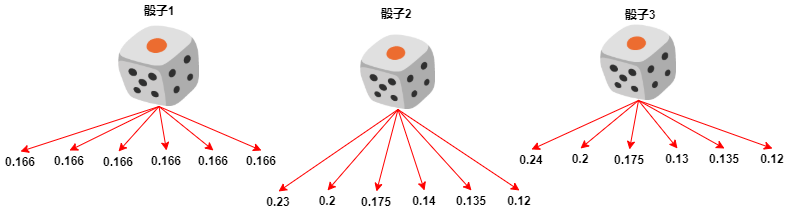• 开始，随机从色子中选取一个色子，进行投掷，记录其点数后放回，等待下一次投掷；

• 然后，再选择一个色子。选取的规则是：

• 若当前投掷的色子是骰子1，那么下一次一定拿骰子2
• 若当前投掷的色子是骰子2，那么下一次各个骰子选择的概率分别为(left( 0.2,0.35,0.45 ight))
• 若当前投掷的色子是骰子3，那么下一次各个骰子选择的概率分别为(left( 0.4,0.14,0.46 ight))
• 确定使用的色子后，再次进行投掷，记录其点数后，放回；

• 重复上面的操作过程6次，得到一个观测序列：

(O=left( 6,3,1,2,4,2 ight))

​ 整个过程玩家只能看见色子的点数，看不见是否更换色子。

​ 这个案例中，有两个随机序列。第一个是骰子的序列；第二个是骰子投掷的点数序列。第一个序列是隐藏的，后者是可见的。这就是一个简单的隐马尔可夫案例。

## 2.2 案例的主要参数

​ 根据前面的案例说明，下面我们梳理出，骰子造假案的主要参数。

]

]

[Pi= {left( frac{1}{3},frac{1}{3},frac{1}{3} ight)}’
]

[A=egin{bmatrix}
0 & 1 & 0\
0.2 & 0.35 & 0.45\
0.4 & 0.14 & 0.46
end{bmatrix}
]

[B=egin{bmatrix}
frac{1}{6} & frac{1}{6} & frac{1}{6} & frac{1}{6} & frac{1}{6} & frac{1}{6}\
0.23 & 0.2 & 0.175 & 0.14 & 0.135 & 0.12\
0.24 & 0.2 & 0.175 & 0.13 & 0.135 & 0.12
end{bmatrix}
]

# 3、隐马尔可夫的三个基本问题

​ 经过第一章与第二章，我们对隐马尔可夫的观测序列生成应该有大概的了解了。在隐马尔可夫模型中有三个需要解决的问题。下面先分布简介。

# 4、概率计算方法

​ 根据模型假设，对于观测序列，我们可以穷举所有状态序列，但是这里的情况是一个组合排列问题，计算量非常大。计算复杂度为(Oleft( TN^{T} ight))，明显这个复杂度是很大的，不适合我们用于计算较长、复杂的隐马尔可夫模型，下面将介绍著名的forward-backward algorithm

## 4.1 前向算法

[alpha_{t} left( i ight) = P left( o_{1},o_{2},cdots,o_{t},i_{t}=q_{i}|lambda ight)
]

​ 当我们知道时刻(t)的所有前向概率分布，则计算(t+1)时刻就非常简单了。对于某个状态(q_{i})生成某一个观测，我们只需要从时刻(t)所有状态分别乘以此状态转移到目标状态(q_{i})的转移概率，再乘以观测出现的概率，最后求和，这样就可以得到在(t+1)时刻的状态(q_{i})的前向概率。

​ 随着时刻(t)的推进，最终我们可以直接求得(Pleft ( O|lambda ight )=sum_{i=1}^{N}alpha_{T}left ( i ight ))

• 根据初始化求第一次投掷色子的前向概率分布；
• 第二次取状态(q_{i})，产生观测(o_{t})的前向概率可以有状态转移矩阵与观测概率矩阵求得；
• 则第二次投掷的前置概率分布可以由第二步类似求得；
• 递归上面的2、3步可求(t)时刻的前置概率分布；
• 最后可以求得(Pleft ( O|lambda ight )=sum_{i=1}^{N}alpha_{T}left ( i ight ))

• 初始值

(alpha_{1} left ( i ight )=pi _{i}b_{i}left ( o_{1} ight ), quad i=1,2,cdots ,N)

• 递归(t=1,2,cdots,T-1)

(alpha_{t+1}left ( i ight )=left [ sum_{j=1}^{N}alpha_{t}left ( j ight )alpha_{ji} ight ]b_{i}left ( o_{t+1} ight ),quad i=1,2,cdots ,N)

• 终止

(Pleft ( O|lambda ight )=sum_{i=1}^{N}alpha_{T}left ( i ight ))

​ 上面我从三个角度进行了解释，对前向概率的计算应该比较清楚了，下面给出插图方便理解。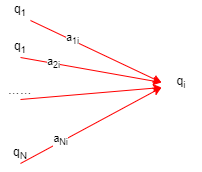## 4.2 前向概率案例计算

### 4.2.1 数值计算

[alpha_{1} left ( 1 ight )=pi_{1}b_{1}left ( o_{1} ight )=0.0556\
alpha_{1} left ( 2 ight )=pi_{2}b_{2}left ( o_{1} ight )=0.0400\
alpha_{1} left ( 3 ight )=pi_{3}b_{3}left ( o_{1} ight )=0.0400
]

[alpha_{2} left ( 1 ight )=left [ sum_{i=1}^{3}alpha_{1}left ( i ight ) a_{i1} ight ]b_{1}left ( o_{2} ight )=left(0.0556 imes 0+0.0400 imes0.2+0.0400 imes0.4 ight) imes frac{1}{6}=0.0040\
alpha_{2} left ( 2 ight )=left [ sum_{i=1}^{3}alpha_{1}left ( i ight ) a_{i2} ight ]b_{2}left ( o_{2} ight )=left(0.0556 imes 1+0.0400 imes0.35+0.0400 imes0.14 ight) imes 0.175=0.0132\
alpha_{2} left ( 3 ight )=left [ sum_{i=1}^{3}alpha_{1}left ( i ight ) a_{i3} ight ]b_{3}left ( o_{2} ight )=left(0.0556 imes 0+0.0400 imes0.45+0.0400 imes0.46 ight) imes 0.175=0.0064\
]

[alpha_{t+1} = left( alpha_{t} imes A ight) * {B[:,index(o_{t+1})]}’
]

[Pleft ( O|lambda ight )=sum_{i=1}^{6}alpha_{6}left ( i ight )=0.0000273
]

### 4.2.2 代码实现

``````import numpy as np

A = np.array([
[0, 1, 0],
[0.2, 0.35, 0.45],
[0.4, 0.14, 0.46]
])
B = np.array([
[1 / 6, 1 / 6, 1 / 6, 1 / 6, 1 / 6, 1 / 6],
[0.23, 0.2, 0.175, 0.14, 0.135, 0.12],
[0.24, 0.2, 0.175, 0.13, 0.135, 0.12]
])

class HMM(object):
"""
隐马尔可夫模型
:param transformer: 状态转移概率矩阵
:param observation: 观测概率矩阵
:param pi: 初始状态分布
"""

def __init__(self, transformer, observation, pi):
self.transformer = transformer
self.observation = observation
self.pi = pi

def forward_prob(self, last_forward, observation):
"""
计算时刻t的前向概率分布
:param last_forward: 上一时刻的概率分布
:param observation: 观测概率向量
:return: 当前的前向概率分布
"""
res = np.dot(last_forward, self.transformer) * observation
return res

def forward_prob_distribution(self, observe_seq):
"""
计算观测序列出现的概率
:param observe_seq: 观测序列
:return: 前向概率分布
"""
last_prob = self.pi * self.observation[:, observe_seq - 1]
for v in observe_seq[1:]:
last_prob = self.forward_prob(last_prob, self.observation[:, v - 1])
return last_prob

def get_forward_prob(self, observe_seq):
"""
计算观测序列出现的概率
:param observe_seq: 观测序列
:return: 观测序列的概率值
"""
last_prob = self.forward_prob_distribution(observe_seq)
return np.sum(last_prob)

if __name__ == '__main__':
hmm = HMM(A, B, [1/3, 1/3, 1/3])

print(result)

``````

``````A = np.array([
[0.5, 0.2, 0.3],
[0.3, 0.5, 0.2],
[0.2, 0.3, 0.5]
])
B = np.array([
[0.5, 0.5],
[0.4, 0.6],
[0.7, 0.3]
])
result = hmm.get_forward_prob([6, 3, 1, 2, 4, 2])
hmm = HMM(A, B, [0.2, 0.4, 0.4])
result = hmm.get_forward_prob([1, 2, 1])
``````

## 4.3 后向算法

[eta_{t}left ( i ight )=Pleft ( o_{t+1},o_{t+2},cdots ,o_{T} |i_{t}=q_{i},lambda ight )
]

• 初始值

[eta_{T}left ( i ight )=1,quad i=1,2,cdots ,N
]

• 递归：(t=T-1,T-2,cdots,1)

[eta_{t}left ( i ight )=sum_{j=1}^{N}a_{ij}b_{j}left ( o_{t+1} ight )eta_{t+1}left ( j ight ),quad i=1,2,cdots ,N
]

• 终止：

[Pleft ( O|lambda ight )=sum_{j=1}^{N}pi_{i}b_{i}left ( o_{1} ight )eta_{1}left ( i ight )
]

## 4.4 后向概率案例计算

[eta_{t} = A imes left(B[:,index(o_{t+1})] * eta_{t+1}ight)
]

### 4.4.1 代码实现

``````def backward_prob(self, last_backward, observation):
"""
计算时刻t的后向概率分布
:param last_backward: 下一时刻的概率分布
:param observation: 观测概率向量
:return: 当前的后向概率分布
"""
return np.dot(self.transformer, observation * last_backward)

def backward_prob_distribution(self, observe_seq):
"""
计算观测序列出现的概率
:param observe_seq: 观测序列
:return: 后向概率分布
"""
last_backward = np.array( * self.transformer.shape)
observe_seq.reverse()
for v in observe_seq[:-1]:
last_backward = self.backward_prob(last_backward, self.observation[:, v - 1])
return last_backward

def get_backward_prob(self, observe_seq):
"""
计算观测序列出现的概率
:param observe_seq: 观测序列
:return: 观测序列的概率值
"""
last_backward = self.backward_prob_distribution(observe_seq)
return np.sum(self.pi * self.observation[:, observe_seq[-1] - 1] * last_backward)
``````

### 4.4.2 代码测试

``````if __name__ == '__main__':
hmm = HMM(A, B, [1/3, 1/3, 1/3])
result = hmm.get_forward_prob([6, 3, 1, 2, 4, 2])
print(result)
result = hmm.get_backward_prob([6, 3, 1, 2, 4, 2])
print(result)
``````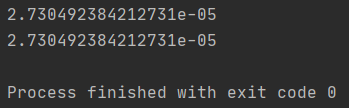``````if __name__ == '__main__':
# A B换成书上的相应矩阵
hmm = HMM(A, B, [0.2, 0.4, 0.4])
result = hmm.get_forward_prob([1, 2, 1])
print(result)
result = hmm.get_backward_prob([1, 2, 1])
print(result)
``````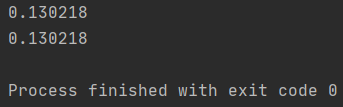## 4.5 前向–后向概率计算方法

[Pleft ( O|lambda ight )=sum_{i=1}^{N}sum_{j=1}^{N}alpha_{t}left ( i ight )a_{ij}b_{j}left ( o_{t+1} ight )eta_{t+1}left ( j ight ), quad t=1,2,cdots ,T-1
]

### 4.5.1 前向–后向计算代码

``````def get_forward_backward_prob(self, observe_seq, split_index=1):
"""
同时使用前向--后向概率计算观测的条件概率
:param observe_seq: 观测序列
:param split_index: 切割的时刻, 这个时刻默认分配给前向算法
:return: 观测序列的条件概率
"""
assert split_index < len(observe_seq),
"Expect split_index to be less than the length of observe_seq."
forward_distribution = self.forward_prob_distribution(observe_seq[:split_index])
backward_distribution = self.backward_prob_distribution(observe_seq[split_index:])
res = np.dot(
forward_distribution,
np.dot(self.transformer,
self.observation[:, observe_seq[split_index] - 1] * backward_distribution)
)
return res
``````

### 4.5.2 代码验证

``````if __name__ == '__main__':
hmm = HMM(A, B, [1/3, 1/3, 1/3])
result = hmm.get_forward_prob([6, 3, 1, 2, 4, 2])
print(result)
result = hmm.get_backward_prob([6, 3, 1, 2, 4, 2])
print(result)
result = hmm.get_forward_backward_prob([6, 3, 1, 2, 4, 2], 3)
print(result)
``````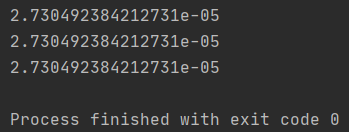## 4.6 一些概率与期望值的计算

​ 利用前向概率、后向概率可以获取关于单个状态和两个状态概率的计算公式。

### 4.6.1 给定观测(t)时刻状态为(q_{i})的概率

​ 对于给定的模型(lambda) 和观测序列(O)，在时刻(t)处于状态(q_{i})的概率为：

[gamma_{t}left ( i ight )=Pleft ( i_{t}=q_{i}|O,lambda ight )=frac{Pleft ( i_{t}=q_{i},O|lambda ight )}{Pleft ( O|lambda ight )}
]

[alpha_{t}(i)eta_{t}(i)=Pleft ( i_{t}=q_{i},O|lambda ight )
]

[gamma_{t}left ( i ight )=frac{alpha_{t}(i)eta_{t}(i)}{sum_{j=1}^{N}alpha_{t}(j)eta_{t}(j)}
]

``````def get_qi2t_prob(self, observe_seq, t, qi):
"""
对于给定隐马尔可夫模型与观测序列，计算在时刻t处于状态qi的概率
:param observe_seq: 观测序列
:param t: 时刻的编码, [默认从1开始编码]
:param qi: 状态的编码, [默认从1开始编码]
:return: 概率值
"""
forward_distribution = self.forward_prob_distribution(observe_seq[:t])
backward_distribution = self.backward_prob_distribution(observe_seq[t-1:])
res = forward_distribution[qi-1] * backward_distribution[qi-1]
/ np.dot(forward_distribution, backward_distribution)
return res
``````

``````result = hmm.get_qi2t_prob([6, 3, 1, 2, 4, 2], 3, 2)
print(result)
Out:
0.427265264306416
``````

### 4.6.2 给定观测时刻(t)状态为(q_{i}),时刻(t+1)为(q_{j})的概率

​ 对于给定的模型(lambda) 和观测序列(O)，在时刻(t)处于状态(q_{i})，且下一时刻为(q_{j})的概率为：

[xi _{t}left ( i,j ight )=Pleft ( i_{t}=q_{i},i_{t+1}=q_{j}|O,lambda ight )=frac{Pleft ( i_{t}=q_{i},i_{t+1}=q_{j},O|lambda ight )}{Pleft ( O|lambda ight )}
]

[Pleft ( i_{t}=q_{i},i_{t+1}=q_{j},O|lambda ight )=alpha_{t}(i)a_{ij}b_{j}left( o_{t+1} ight)eta_{t+1}(j)
]

``````def get_qi2t_qj2next_prob(self, observe_seq, t, qi, qj):
"""
对于给定隐马尔可夫模型与观测序列，计算在时刻t处于状态qi,且下一时刻处于qj状态的概率
:param observe_seq: 观测序列
:param t: 时刻的编码, [默认从1开始编码]
:param qi: t时刻状态的编码, [默认从1开始编码]
:param qj: t+1时刻状态的编码, [默认从1开始编码]
:return: 概率值
"""
forward_distribution = self.forward_prob_distribution(observe_seq[:t])
backward_distribution = self.backward_prob_distribution(observe_seq[t:])
denominator = np.dot(
forward_distribution,
np.dot(self.transformer,
self.observation[:, observe_seq[t] - 1] * backward_distribution)
)
numerator = forward_distribution[qi - 1] * self.transformer[qi - 1, qj - 1] * self.observation[
qj - 1, observe_seq[t] - 1] * backward_distribution[qj - 1]
return numerator / denominator
``````

### 4.6.3 给定观测下出现状态(i)的期望

[1 – prod_{t=1}^{T} left( 1 – gamma_{t}left ( i ight ) ight )
]

``````def expect_i_appear_trans(self, observe_seq, qi, transfer=False):
"""
在观测O下, 计算状态qi出现的概率
:param observe_seq: 观测序列
:param qi: 状态的编码, [默认从1开始编码]
:param transfer: 是否由状态qi转移, 默认False
:return: 概率值
"""
res = 1
for t in range(1, len(observe_seq)):
res *= 1 - self.get_qi2t_prob(observe_seq, t, qi)
if not transfer:
res *= 1 - self.get_qi2t_prob(observe_seq, len(observe_seq), qi)
return 1 - res
``````

​ 这一节的公式在书本上是直接相加，我们回顾一下对于给定的模型(lambda) 和观测序列(O)，在时刻(t)处于状态(q_{i})的概率为(gamma_{t}left ( i ight ))，在(t)时刻处于状态(q_{i})，这个事件中可能包括了其他时刻也处于状态(q_{i})。如果我们直接对时刻进行求和，出现的概率就会重复计算，导致出现超过1的期望值。

### 4.6.4 给定观测下由状态(i)转移的期望

[1 – prod_{t=1}^{T-1} left( 1 – gamma_{t}left ( i ight ) ight )
]

``````A = np.array([
[0, 1, 0],
[0.2, 0.35, 0.45],
[0.4, 0.14, 0.46]
])
B = np.array([
[1 / 6, 1 / 6, 1 / 6, 1 / 6, 1 / 6, 1 / 6],
[0.23, 0.2, 0.175, 0.14, 0.135, 0.12],
[0.24, 0.2, 0.175, 0.13, 0.135, 0.12]
])
hmm = HMM(A, B, [1 / 3, 1 / 3, 1 / 3])
result = hmm.expect_i_appear_trans([6, 3, 1, 2, 4, 2], 3, True)
print(result)
------------------------------------------------------------
Out:
0.8718306572257475
------------------------------------------------------------

result = hmm.expect_i_appear_trans([6, 3, 1, 2, 4, 2], 3)
print(result)
------------------------------------------------------------
Out:
0.9155699657722511
------------------------------------------------------------
``````

### 4.6.5 给定观测下由状态(i)转移到状态(j)的期望

[1 – prod_{t=1}^{T-1} left( 1 – xi _{t}left ( i,j ight ) ight )
]

``````def expect_i2j(self, observe_seq, qi, qj):
"""
在观测O下, 计算状态转移的概率
:param observe_seq: 观测序列
:param qi: 状态的编码, [默认从1开始编码]
:param qj: 状态的编码, [默认从1开始编码]
:return: 概率值
"""
res = 1
for t in range(1, len(observe_seq)):
res *= 1 - self.get_qi2t_qj2next_prob(observe_seq, t, qi, qj)
return 1 - res
``````

``````A = np.array([
[0, 1, 0],
[0.2, 0.35, 0.45],
[0.4, 0.14, 0.46]
])
B = np.array([
[1 / 6, 1 / 6, 1 / 6, 1 / 6, 1 / 6, 1 / 6],
[0.23, 0.2, 0.175, 0.14, 0.135, 0.12],
[0.24, 0.2, 0.175, 0.13, 0.135, 0.12]
])
hmm = HMM(A, B, [1 / 3, 1 / 3, 1 / 3])
result = hmm.expect_i2j([6, 3, 1, 2, 4, 2], 2, 3)
print(result)
------------------------------------------------------------
Out:
0.6501034848435623
------------------------------------------------------------
``````

# 5、隐马尔可夫的学习问题

​ 已知观测序列 (O = left{ o_{1},o_{2},cdots,o_{T} ight})的集合估计模型 (lambda = left( A,B,Pi ight)) 参数，使得在该模型下观测序列的条件概率(P left( O|lambda ight))最大。也就是说已知观测序列，我们要学习一个隐马尔可夫模型，主要是要评估出三个参数(A,B,Pi)，使得当前事件发生的概率是最大的，明显这就是极大似然。关于学习方法，我们主要叙述：极大似然估计、Baum-Welch算法(鲍姆·韦尔奇算法；实际就是EM算法)。

## 5.2 鲍姆·韦尔奇算法

​ 首先回顾EM算法，简单来说就是对参数求期望，再对期望取最大值，如此循环直到模型收敛。

[Pleft ( O |lambda ight ) = sum_{I}Pleft ( O|I,lambda ight )Pleft ( I|lambda ight )
]

HMM结构示意图：### 5.2.1 引入目标函数

​ 这里我们还是要使用到极大似然的思想，目标函数以对数似然函数为对象。

• 观测数据：(O = left{ o_{1},o_{2},cdots,o_{T} ight})
• 隐藏数据：(I = left{ i_{1},i_{2},cdots,i_{T} ight})
• 完全数据(left(O,I ight) = left{ o_{1},o_{2},cdots,o_{T},i_{1},i_{2},cdots,i_{T} ight})
• 目标函数： \$ ext{log}P left( O,I| lambda ight)\$

### 5.2.2 EM算法的E步：求Q函数

[Qleft(lambda,ar{lambda} ight)=
sum_{I} ext{log}Pleft(O,I|lambda ight)*Pleft(O,I|ar{lambda} ight)
]

[Pleft ( O |lambda ight ) =
pi_{i_{1}}b_{i_{1}}left ( o_{1} ight )a_{i_{1}i_{2}}b_{i_{2}}left ( o_{2} ight )
cdots
a_{i_{T-1}i_{T}}b_{i_{T}}left ( o_{T} ight )
]

Q函数可以写成

[Qleft(lambda,ar{lambda} ight)=
sum_{I} ext{log}\,pi_{i_{1}} * Pleft(O,I|ar{lambda} ight) +
sum_{I} left(sum_{t=1}^{T-1} ext{log}\,a_{i_{t}i_{t+1}} ight) *Pleft(O,I|ar{lambda} ight) +
sum_{I} left(sum_{t=1}^{T} ext{log}\,b_{i_{t}}left ( o_{t} ight ) ight) *Pleft(O,I|ar{lambda} ight)
]

(29)式是将式(28)带入式(27)，把( ext{log})里面的部分拆开。上式明显被分为了三部分，第一部分是关于初始状态概率分布；第二部分是状态转移概率矩阵元素；第三部分是关于观测概率矩阵元素。这正好对应了隐马尔可夫模型的三个参数。

#### 5.2.2.1 E步总结

• 首先，我们看(Pleft(O,I|ar{lambda} ight))表示什么？这个概率表示在当前模型估计下，观测状态(O)对应的隐状态为(I)的概率。
• 其次，我们思考( ext{log}P left( O,I| lambda ight))表示什么？这个值难道不是表示我们在模型(lambda)下，观测观测状态(O)对应的隐状态为(I)的对数似然概率嘛，也即这个值表示了我们目标函数的一个取值。
• 最后，我们看求和符号。将所有取值乘以其出现概率再求和，这不就是期望吗？这里的期望是对数似然的期望！

### 5.2.3 EM算法的M步：求一组参数(A,B,Pi)极大化期望

#### 5.2.3.1 初始状态分布的M步估计

[sum_{I} ext{log}\,pi_{i_{1}} * Pleft(O,I|ar{lambda} ight) =
sum_{i=1}^{N} ext{log}\,pi_{i} * Pleft(O,i_{1}=i|ar{lambda} ight)
]

[sum_{i=1}^{N} ext{log}\,pi_{i} * Pleft(O,i_{1}=i|ar{lambda} ight) + gammaleft(sum_{i=1}^{N}pi_{i} -1 ight)
]

​ 那么求导就得到法线了？是的，求导就可以得到法线。对于一个一般函数(f(x_{1},x_{2},cdots,x_{l}))，则它的法向量可以表示为：(left [ frac{partial f}{partial x_{1}}, frac{partial f}{partial x_{1}},cdots , frac{partial f}{partial x_{l}} ight ])

[frac{partial }{partial pi_{i}}left [sum_{i=1}^{N} ext{log}\,pi_{i} * Pleft(O,i_{1}=i|ar{lambda} ight) + gammaleft(sum_{i=1}^{N}pi_{i} -1 ight) ight ]=0\
Pleft(O,i_{1}=i|ar{lambda} ight) + gamma pi_{i} =0\
Rightarrow gamma =-Pleft(O|ar{lambda} ight)
]

[pi_{i}=frac{Pleft(O,i_{1}=i|ar{lambda} ight)}{Pleft(O|ar{lambda} ight)}
]

#### 5.2.3.2 状态概率转移矩阵的M步估计

[sum_{I} left(sum_{t=1}^{T-1} ext{log}\,a_{i_{t}i_{t+1}} ight) *Pleft(O,I|ar{lambda} ight)=

sum_{i=1}^{N} sum_{j=1}^{N} sum_{t=1}^{T-1} ext{log}\,a_{ij} *Pleft(O,i_{t}=i,i_{t+1}=j|ar{lambda} ight)
]

[frac{partial }{partial a_{ij}} left[sum_{i=1}^{N} sum_{j=1}^{N} sum_{t=1}^{T-1} ext{log}\,a_{ij} *Pleft(O,i_{t}=i,i_{t+1}=j|ar{lambda} ight) + gammasum_{i=1}^{N}left( sum_{j=1}^{N}a_{ij} -1 ight) ight] = 0\

sum_{t=1}^{T-1} Pleft(O,i_{t}=i,i_{t+1}=j|ar{lambda} ight) + gamma a_{ij} = 0\

Rightarrow gamma = -sum_{t=1}^{T-1} Pleft(O,i_{t}=i|ar{lambda} ight)
]

[a_{ij} = frac{sum_{t=1}^{T-1} Pleft(O,i_{t}=i,i_{t+1}=j|ar{lambda} ight)}{sum_{t=1}^{T-1} Pleft(O,i_{t}=i|ar{lambda} ight)}
]

#### 5.2.3.3 观测概率矩阵的M步估计

[sum_{I} left(sum_{t=1}^{T} ext{log}\,b_{i_{t}}left ( o_{t} ight ) ight) *Pleft(O,I|ar{lambda} ight) = sum_{j=1}^{N} sum_{t=1}^{T} ext{log}b_{j}left( o_{t} ight) Pleft( O,i_{t}=j | ar{lambda}ight)
]

[frac{partial }{partial b_{j}left( k ight)} left[ sum_{j=1}^{N} sum_{t=1}^{T} ext{log}b_{j}left( o_{t} ight) Pleft( O,i_{t}=j | ar{lambda}ight) + gamma sum_{j=1}^{N} left( sum_{k=1}^{M}b_{j}left( k ight)-1 ight) ight] = 0 \

sum_{t=1}^{T}Pleft( O,i_{t}=j | ar{lambda}ight) mathbb{I}left(o_{t}=k ight) + gamma b_{j}left( k ight) = 0\

Rightarrow gamma=-sum_{t=1}^{T}Pleft( O,i_{t}=j | ar{lambda}ight)
]

[b_{j}left( k ight) = frac{sum_{t=1}^{T}Pleft( O,i_{t}=j | ar{lambda}ight) mathbb{I}left(o_{t}=k ight)}{sum_{t=1}^{T}Pleft( O,i_{t}=j | ar{lambda}ight)}
]

### 5.2.4 Baum-Welch算法

• 初始化参数(A,B,Pi)
• 按照5.2.2求E步
• 按照5.2.3求M步
• 重复第二、三步，知道参数(A,B,Pi)收敛